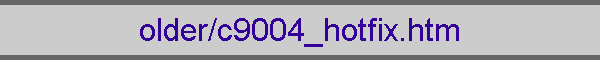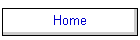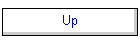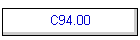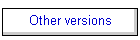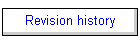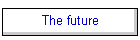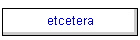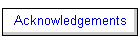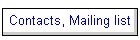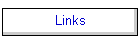# Hot Fixes to C90.04

These are small changes that will be incorporated in the next revision of the code. These only apply to the current version of the code stored on this site. In the following, the corrections that should be made to version 90.04 are indicated by line numbers within specific subroutines.Better fits to Shull and van Steenberg iron radiative recombination rate coefficients.  Their rate coefficients do not have the correct low and high temperature behavior.  Dima Verner has refit them with the correct formula.  Simply replace the existing version of rrfit.f with the new version on Dima Verner's web page, and delete the existing radrec.f (the old rrfit.f and radrec.f are now combined into the one file).   Thanks for Daniel Savin for pointing out this problem with the Shull and van Steenberg fits, and to Kirk Korista for debugging the instructions.  1999 June 30Photoionization rate of some non-existent shells computed incorrectly.  The code treats each shell of every ion separately (see figure 2 of PASP 110, 761).  There are a few ions where Opacity Project photoionization calculations show that strong electron correlations prevent distinct shells from being identified.  In these cases the two shells are treated as a single shell, with the missing shell signaled by placing the pointer to the lower threshold to an energy higher than the higher threshold.  One photoionization rate service routine did not protect against this possibility, resulting in large (incorrect) photoionization rates for especially intense FIR continua.

Edit routine gamk.f and add the test for the two limits.  Change the code around line 69 to read as follows:

```*     >>chng 96 sept 26, remove check on zero opacity, for partial shells
*     >>chng 99 Apr 02, above change introduced problem with non-existent
*     shells, such as 3d of ca+.  There are indicated by setting upper
*     limit below lower limit.  This test was missing, causing incorrect
*     photoionization rate for intense radio continua.
if(n1 .ge. nflux .or. n1.ge.n2 ) then
secion = 0.
secla = 0.
gamk = 0.
h = 0.
return
endif```

This bug had no effect for most continua (and so escaped detection), but could strongly affect the ionization of K and Ca if the FIR continuum was especially intense.  Many thanks for Mark Giroux for uncovering this problem.  1999 April 02.Local emissivity diffuse continua returned by punch lines structure command is incorrect.  The code tracks the local emissivity and total intensity for emission lines by integrating over the first quantity to get the second.  The is formally correct for the lines since they are transferred using escape probabilities.  Diffuse continua are transferred by other methods, and so the local observed emissivity is not really a defined quantity if the continuum is optically thick at that energy.

This problem affected the set of predicted diffuse continua that are punched with the "punch lines structure" command.  The numbers are correct.   The problem is with the local emissivity - this is basically a random number before the following fix.  The problem only affected the output from the "punch lines structure" command, or returned from the cdEms routine if the code is driven as a subroutine.

To correct this problem edit routine lineset1.f, and go to the loop that begins on line 702.  Edit this loop to appear as follows.  Comments before the three new lines of Fortran begin with *>>>> and there are no other changes other than the six new lines.

```*     predict emitted continuum at series of continuum points
do i=1,nPredCont
*       reflected toal continuum (dif+incident emitted inward direction)
ConRef = refcon(ipPredCont(i))/widflx(ipPredCont(i))*
1  anu2(ipPredCont(i))*en1ryd
*       reflected part of INCIDENT continuum (only incident)
ConIn = reflux(ipPredCont(i))/widflx(ipPredCont(i))*
1  anu2(ipPredCont(i))*en1ryd
*       outward continuum
ConOt = outcon(ipPredCont(i))/widflx(ipPredCont(i))*
1  anu2(ipPredCont(i))*en1ryd*r1r0sq
*       this is wavelength in Angstroms, energies stored in EnrPredCont
low = rydlam / EnrPredCont(i)
*       some are hundreds of microns, overflows in printout, cnvrt to microns
if( low.gt. 100 000 ) low = low / 10 000
sumlin(nsum+1) = 0.
call linadd( (ConRef+ConOt)/dVeff, low , 'nFnu' ,'i')
*>>>>>>>change to get correct emissivity
emslin(nsum) = DiffCont(ipPredCont(i))/widflx(ipPredCont(i))*
1  anu2(ipPredCont(i))*en1ryd
sumlin(nsum+1) = 0.
*       total reflected continuum, total inward emission plus
*       reflected diffuse continuum
call linadd( ConRef        /dVeff, low , 'InwT' ,'i')
*>>>>>>>change to zero this emissivity
emslin(nsum) = 0.
sumlin(nsum+1) = 0.
*       reflected incident continuum (only incident)
call linadd( ConIn         /dVeff, low , 'InwC' ,'i')
*>>>>>>>change to zero this emissivity
emslin(nsum) = 0.
*	write(qq,'(i6)') low
end do```

Many thanks to Jao Wei-Chun and Mark Shure for uncovering this problem.   1999 Mar 30.Incorrect format for punch pressure output.  Edit routine dopunch.f, and change line 1066 to read as follows (change the 6e10.2 to 7e10.2):

```        else if( chPunch(i).eq.'PRES' ) then
write(ipPnunit(i),'(1x,1p,7e10.2,l3)') depth, pnow,
2    pinteg-pinzon, lgPresOK```

Thanks for Sabine Philipp for discovering this problem.  1999 Mar 26.Collision strengths for [Cl IV] were set with incorrect variables.    edit routine clcol, and change lines 219 through 225 to read as follows (three changes):

```* >>refer Galavis, M.E., Mendoza, C., & Zeippen, C.J. 1995, A&AS, 111, 347
a21 = 0.251
cs12 = 6.437
a32 = 2.80
*>>>hotfix >>chng 99 jan 20, cs 23 and 13 were swapped around
*following swapped around
cs23 = min(2.1, 0.0450 * te30*te03*te03)
a31 = 2.50
*following swapped around
cs13 = 1.922
* POP3(G1,G2,G3,O12,O13,O23,A21,A31,A32,E12,E23,P2,ABUND,GAM2)```

The declared variables cs32 and cs31 are no longer used and may be removed, although they will do no harm.  This problem had existed since roughly 1992, and passed through many Fortran compilers unnoticed.  It was converted to C along with the rest of the code, and was automatically detected by PCLint.  1999 Jan 20Outwardly transmitted lines not properly added to continua for some printer plot, print commands, and punch commands.  The most significant was the punch transmitted continuum command.  To correct this edit routine dopunch.f and go to line 757.  It will read as follows:

```       conem = (ContDiff(j)+outcon(j))/widflx(j)*anu2(j) +
1 outlin(j)*anu(j)```

This should be changed to read

```        conem = (ContDiff(j)+outcon(j)+outlin(j))/widflx(j)*
1  anu2(j)```

The other commands affected were the plot continuum commands, which are used to produce very rough plots within the output, and the print continuum command, which is no longer in active use.   Thanks for Peter van Hoof for discovering this problem.  1998 Sep 22.The HeI 2.06mm line.  Bob Blum noticed that C90 predicts this line to have an intensity about 2-3 times different than C84 did.  I investigated this difference and wrote a short note describing the causes.  It is in ApJ 512, 247.  Here is how to make the small change to go over to the approximations recommended in that paper:

Edit routine hetran.f and go to line 256.  Change the 'INCO' to ' K2' (there are two spaces between the first single quote and the K, and the K must be capitalized). It will then use Hummer 1968 instead of the more limited Hummer and Kurucz 1980. The results do change for some parameters.  1998 Aug 15.  Thanks to Bob Blum for discovering that the predicted intensity of this line had changed.Necessary code commented out in fabden. FABDEN generates local hydrogen densities that can be a function of anything.  It is involved with the dlaw command, which can have numbers on the command line.  These numbers are passed to fabden in common block dlaw.  The contents of this array can be used to generate the density.  This common block is commented out in the source.  Edit the routine and change line 6 from

`*     include "dlaw.com"`

to

```      double precision dlaw
*     parameters passed to the dlaw command
common/dlaw/ dlaw(10)
```

With this change the description of fabden in hazy will be correct.   1998 Feb 27.  Thanks to Dick Henry for uncovering this problem.Numerical instability in H2 population and cooling in some PDR calculations.  The solution is to avoid canceling two very large exponentials.  Edit routine hmole.f.  On line 421change the temperature in the exponential from 2.12e4 to the more accurate 2.123e4.  It will then read

`      rh2h2p = 1.8e-12 * sqrte*te10/te01* sexp(2.123e4/te)`

Now add the following two lines to just after this statement: (these will now be lines 422 and 423 of hmole).

```*     inverse rate
bh2h2p = 1.8e-12 * sqrte*te10/te01*2./16.```

Finally delete lines 599 through 605 of the revised file.  These line were the following:

```*     inverse rate
if( phplte.gt. 0. ) then
*       extra factor of HII appeared before version 85.23, typo!
bh2h2p = rh2h2p * ph2lte/phplte
else
bh2h2p = 0.
endif```

The direct evaluation of bh2h2p is more stable than the use of the ratio of the two LTE population densities.  1998 Jan 2.Crash on Ultra Sparc for very low temperature models.   The code has not been extensively tested on Ultra Sparcs, although it has been developed on a Sparc 20.  Some details of the floating point handling appear to be different on the two CPUs.  In particular the code crashed in routine level3 for one set of models.  Edit level3.f, go to line 586, where you will see the four lines

```*     fraction that was collisionally excited
t21(ipLnColovTOT) = c12 / r12
t10(ipLnColovTOT) = c01 / r01
t20(ipLnColovTOT) = c02 / r02```

```*
*     fraction that was collisionally excited
if( r12.gt.0. ) then
t21(ipLnColovTOT) = c12 / r12
else
t21(ipLnColovTOT) = 0.
endif
if( r01.gt.0. ) then
t10(ipLnColovTOT) = c01 / r01
else
t10(ipLnColovTOT) = 0.
endif
if( r02.gt.0. ) then
t20(ipLnColovTOT) = c02 / r02
else
t20(ipLnColovTOT) = 0.
endif```

1997 Dec 29.  Thanks to Anton Koekemoer for uncovering this problem.